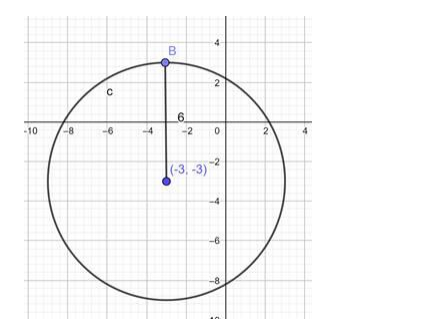# Find the equation of a circle with`
Question:

Find the equation of a circle with Centre $(-3,-2)$ and radius 6

Solution:

The general form of the equation of a circle is:

$(x-h)^{2}+(y-k)^{2}=r^{2}$

Where, (h, k) is the centre of the circle.

r is the radius of the circle.

Substituting the centre and radius of the circle in he general form:

$\Rightarrow(x-(-3))^{2}+(y-(-2))^{2}=6^{2}$

$\Rightarrow(x+3)^{2}+(y+2)^{2}=36$

Ans; equation of a circle with Centre $(-3,-2)$ and radius 6 is:

$\Rightarrow(x+3)^{2}+(y+2)^{2}=36$